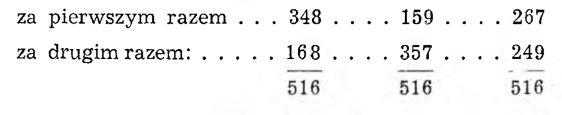# By a strange coincidenceNine digits from 1 do 9 was written on nine pages and distributed to three people. Each person made the smallest possible three-digit number from the three cards they received, it means, that in the place of the hundreds she put the lowest digit she had, in the place of tens - higher, and in the place of unity - the highest. After the numbers were put together, all three people read them aloud and wrote them down. It turned out then, that the sum of the digits of all three numbers was oddly the same. The pages were then mixed up and distributed again in pairs of three. Each of the participants of this game accidentally received one of the previously owned cards and two new ones. And again, by a strange coincidence, the sums of the digits were the same, and what's even more interesting, for each person, the previously arranged number and the now arranged number gave the same sum 516.

What numbers each received on the first and second hands?

The sum of the first nine numbers 1 + 2 + 3 + .. . + 9 = 45. So the sum of the digits on the first and second hands of everyone present had to be 15. The digits could not stand in the place of unity in the numbers sought 1, 2, 3, 4 years 5, because then even the largest three-digit number, which could be arranged according to the given rules (345), gives a total of digits 12.

Sum of numbers (516) ends with 6, so unities in summed numbers had to be represented by 7 i 9 or by 8 i 8. For tens and hundreds, there are numbers from 1 do 6.

But 7 + 9 = 8 + 8 = 16; 516 — 16 = 500. So the sum of the tens we are looking for gives us 0, it means, that the numbers had to come second 4 i 6 or 5 i 5. So the sum of the hundreds is equal to this 4, so they stood in the place of the hundreds 1 i 3 or 2 i 2.

Each person had one digit repeated in both numbers: if it happened again 8, it could only be tens 4 i 6, and hundreds 3 i 1; hence the numbers: 348 i 168. If it happened on the other person 5, they could only be unities 7 i 9, and hundreds 1 i 3; hence the numbers 159 i 357. It happened again in the third person 2, so they were tens 4 i 6, unities 9 i 7; so there were numbers: 267 i 249.

Let's make a statement of numbers: